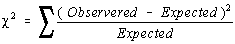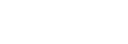# Stats: Test for Independence

In the test for independence, the claim is that the row and column variables are independent of each other. This is the null hypothesis.

The multiplication rule said that if two events were independent, then the probability of both occurring was the product of the probabilities of each occurring. This is key to working the test for independence. If you end up rejecting the null hypothesis, then the assumption must have been wrong and the row and column variable are dependent. Remember, all hypothesis testing is done under the assumption the null hypothesis is true.

The test statistic used is the same as the chi-square goodness-of-fit test. The principle behind the test for independence is the same as the principle behind the goodness-of-fit test. The test for independence is always a right tail test.

In fact, you can think of the test for independence as a goodness-of-fit test where the data is arranged into table form. This table is called a contingency table.The test statistic has a chi-square distribution when the following assumptions are met

• The data are obtained from a random sample
• The expected frequency of each category must be at least 5.

The following are properties of the test for independence

• The data are the observed frequencies.
• The data is arranged into a contingency table.
• The degrees of freedom are the degrees of freedom for the row variable times the degrees of freedom for the column variable. It is not one less than the sample size, it is the product of the two degrees of freedom.
• It is always a right tail test.
• It has a chi-square distribution.
• The expected value is computed by taking the row total times the column total and dividing by the grand total
• The value of the test statistic doesn't change if the order of the rows or columns are switched.
• The value of the test statistic doesn't change if the rows and columns are interchanged (transpose of the matrix)
• The test statistic is## Using the TI-82

There is a program called CONTING (for contingency table) for the TI-82 which will compute the test statistic for you. You still need to look up the critical value in the table.